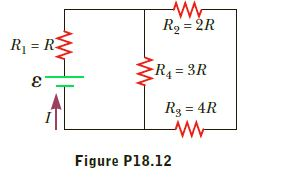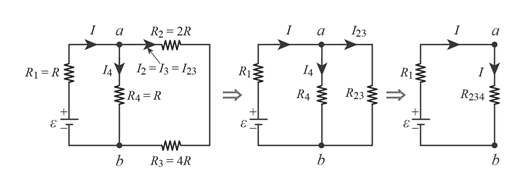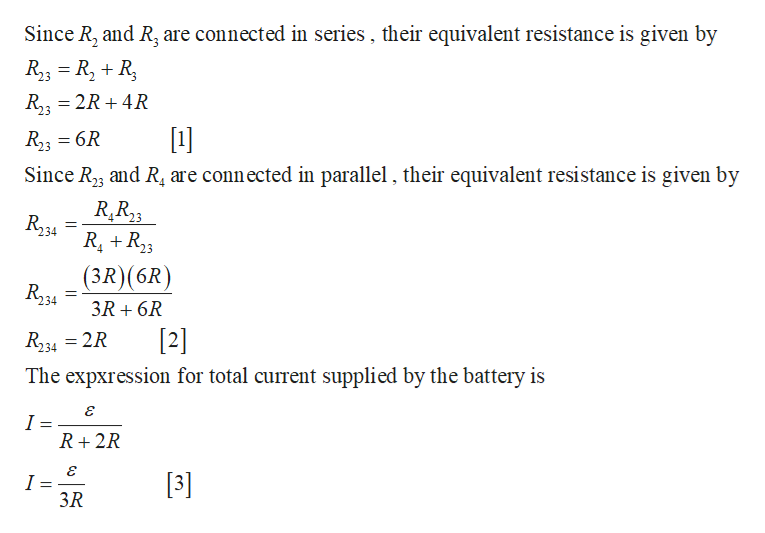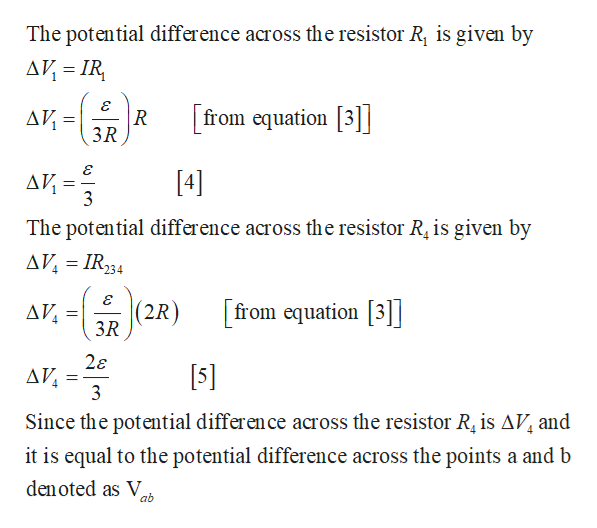Question
1 views

Four resistors are connected
to a battery as shown
in Figure P18.12. (a) Determine
the potential difference
across each resistor in
terms of ε. (b) Determine
the current in each resistor
in terms of I.help_outlineImage TranscriptioncloseR = 2R R = R2 %3D R4 = 3R R3 = 4R Figure P18.12 fullscreen
check_circle

Step 1

The given circuit is reduced in the below figure .help_outlineImage Transcriptionclose123 R2 = 2R I2=13= /23 14 I4 R1 =R R43 R23 = R4 = R R234 ww R3 = 4R ww ww ww ww fullscreen
Step 2help_outlineImage TranscriptioncloseSince R, and R, are connected in series , their equivalent resistance is given by R3 = R, + R, R,; = 2R + 4R 23  R; = 6R Since R23 and R, are connected in parallel , their equivalent resistance is given by -4 R,R23 R34 %3D R, +R23 (3R)(6R) 234 3R + 6R  R234 = 2R The expxression for total current supplied by the battery is R+2R  I = 3R fullscreen
Step 3

Continuing previ...help_outlineImage TranscriptioncloseThe potential difference across the resistor R, is given by AV = IR, AV = 3R from equation || AV =  3 The potential difference across the resistor R, is given by AV, = IR234 (2R) [from equation | AV, = 3R 2ɛ Δν 3  Since the potential difference across the resistor R, is AV, and it is equal to the potential difference across the points a and b den oted as V. ab fullscreen

### Want to see the full answer?

See Solution

#### Want to see this answer and more?

Solutions are written by subject experts who are available 24/7. Questions are typically answered within 1 hour.*

See Solution
*Response times may vary by subject and question.
Tagged in

### Other